## 5.2.3 LP to ISP conversion

26.1903GPPAdaptive Multi-Rate - Wideband (AMR-WB) speech codecSpeech codec speech processing functionsTranscoding functionsTS

The LP filter coefficients ak, k=1,…,16, are converted to the ISP representation for quantization and interpolation purposes. For a 16th order LP filter, the ISPs are defined as the roots of the sum and difference polynomials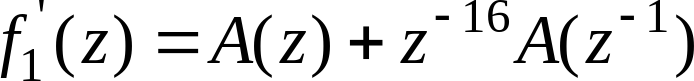( 10 )

and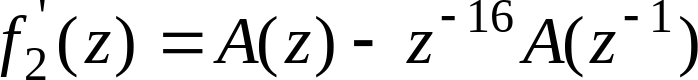( 11 )

respectively. (The polynomials f’1(z) and f’2(z) are symmetric and antisymmetric, respectively). It can be proven that all roots of these polynomials are on the unit circle and they alternate each other . f’2(z) has two roots at z = 1 (=0) and z = -1 (= ). To eliminate these two roots, we define the new polynomials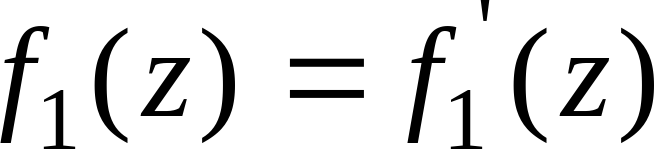( 12 )

and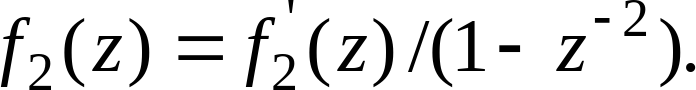( 13 )

Polynomials f1(z) and f2(z) have 8 and 7 conjugate roots on the unit circle respectively. Therefore, the polynomials can be written as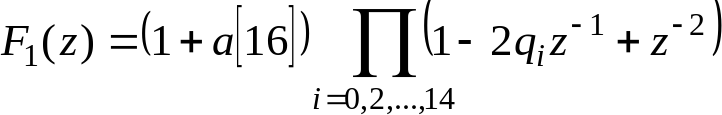( 14 )

and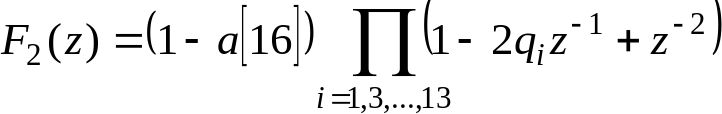( 15 )

where qi=cos(i) with i being the immittance spectral frequencies (ISF) and a is the last predictor coefficient. ISFs satisfy the ordering property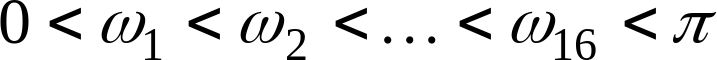. We refer to as the ISPs in the cosine domain.

Since both polynomials f1(z) and f2(z) are symmetric only the first 8 and 7 coefficients of each polynomial, respectively, and the last predictor coefficient need to be computed.

The coefficients of these polynomials are found by the recursive relations

for i=0 to 7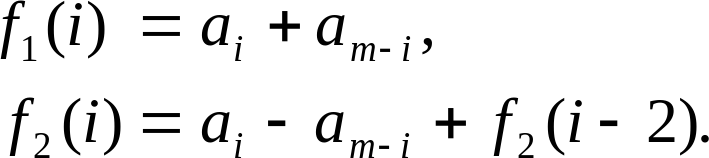( 16 )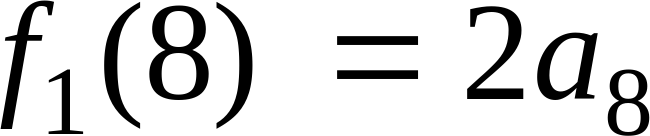where m=16 is the predictor order, and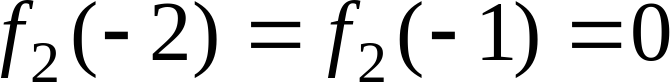.

The ISPs are found by evaluating the polynomials F1(z) and F2(z) at 100 points equally spaced between 0 and and checking for sign changes. A sign change signifies the existence of a root and the sign change interval is then divided 4 times to better track the root. The Chebyshev polynomials are used to evaluate F1(z) and F2(z) . In this method the roots are found directly in the cosine domain {qi}. The polynomials F1(z) and F2(z) evaluated atcan be written as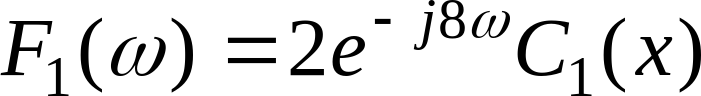and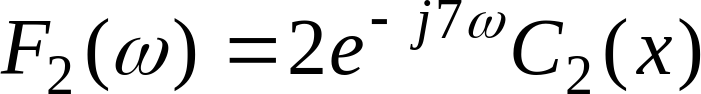( 17 )

with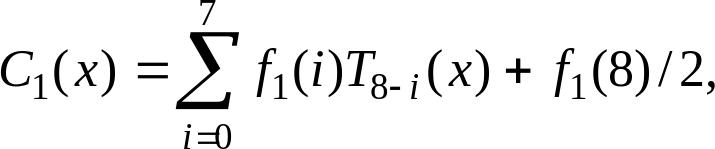and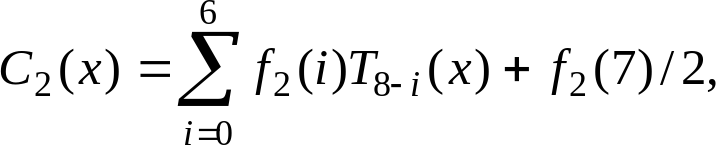( 18 )

where Tm=cos(m) is the mth order Chebyshev polynomial, f(i) are the coefficients of either F1(z) or F2(z), computed using the equations in (16). The polynomial C(x) is evaluated at a certain value of x = cos() using the recursive relation: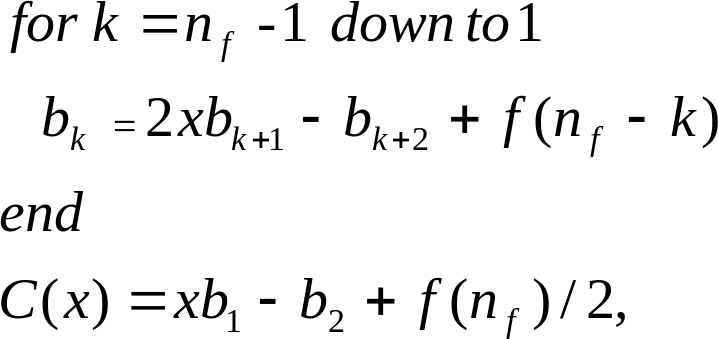where nf=8 in case of C1(x) and nf=7 in case of C2(x), with initial values bnf=f(0) and bnf+1=0. The details of the Chebyshev polynomial evaluation method are found in .